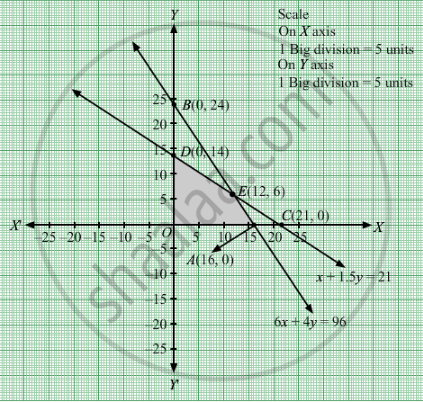# A Library Has to Accommodate Two Different Types of Books on a Shelf. the Books Are 6 Cm and 4 Cm Thick and Weigh 1 Kg and 1 1 2 Kg Each Respectively. - Mathematics

Sum

A library has to accommodate two different types of books on a shelf. The books are 6 cm and 4 cm thick and weigh 1 kg and  $1\frac{1}{2}$ kg each respectively. The shelf is 96 cm long and atmost can support a weight of 21 kg. How should the shelf be filled with the books of two types in order to include the greatest number of books? Make it as an LPP and solve it graphically.

#### Solution

Let x books of first type and y books of second type were accommodated.
Number of books cannot be negative.
Therefore, $x, y \geq 0$

According to question, the given information can be tabulated as:
 Thickness(cm) Weight(kg) First type(x) 6 1 Second type(y) 4 1.5 Capacity of shelf 96 21

Therefore, the constraints are
$6x + 4y \leq 96$

$x + 1 . 5y \leq 21$

Number of books = Z =  $x + y$  which is to be maximised
Thus, the mathematical formulat​ion of the given linear programmimg problem is
MaxImize Z =  $x + y$

subject to

$6x + 4y \leq 96$
$x + 1 . 5y \leq 21$
First we will convert inequations into equations as follows:
6x + 4y = 96, x + 1.5y = 21, x = 0 and y = 0

Region represented by 6x + 4y ≤ 96:
The line 6x + 4y = 96 meets the coordinate axes at A(16, 0) and $B\left( 0, 24 \right)$ respectively. By joining these points we obtain the line
6x + 4y = 96. Clearly (0,0) satisfies the 6x + 4y = 96. So, the region which contains the origin represents the solution set of the inequation
6x + 4y ≤ 96.

Region represented by + 1.5y ≤ 21:
The line x + 1.5y = 21 meets the coordinate axes at C(21, 0) and  $D\left( 0, 14 \right)$ respectively.  By joining these points we obtain the line x + 1.5y = 21. Clearly (0,0) satisfies the inequation + 1.5y ≤ 21. So,the region which contains the origin represents the solution set of the inequation + 1.5y ≤ 21.

Region represented by ≥ 0 and y ≥ 0:
Since, every point in the first quadrant satisfies these inequations. So, the first quadrant is the region represented by the inequations x ≥ 0, and y ≥ 0.
The feasible region determined by the system of constraints  6x + 4y ≤ 96, + 1.5y ≤ 21, x ≥ 0 and y ≥ 0 are as follows.The corner points are O(0, 0), D(0, 14), E(12, 6), A(16, 0)

The values of Z at these corner points are as follows

 Corner point Z= x + y O 0 D 14 E 18 A 16

The maximum value of Z is 18 which is attained at $\left( 12, 6 \right)$

Thus, maximum number of books that can be arranged on shelf is 18 where 12 books are of first type and 6 books are the other type.

Concept: Graphical Method of Solving Linear Programming Problems
Is there an error in this question or solution?

#### APPEARS IN

RD Sharma Class 12 Maths
Chapter 30 Linear programming
Exercise 30.4 | Q 39 | Page 55